# Matrix Completion with Nuclear Norm Minimization¶

Important: Please read the installation page for details about how to install the toolboxes. $\newcommand{\dotp}{\langle #1, #2 \rangle}$ $\newcommand{\enscond}{\lbrace #1, #2 \rbrace}$ $\newcommand{\pd}{ \frac{ \partial #1}{\partial #2} }$ $\newcommand{\umin}{\underset{#1}{\min}\;}$ $\newcommand{\umax}{\underset{#1}{\max}\;}$ $\newcommand{\umin}{\underset{#1}{\min}\;}$ $\newcommand{\uargmin}{\underset{#1}{argmin}\;}$ $\newcommand{\norm}{\|#1\|}$ $\newcommand{\abs}{\left|#1\right|}$ $\newcommand{\choice}{ \left\{ \begin{array}{l} #1 \end{array} \right. }$ $\newcommand{\pa}{\left(#1\right)}$ $\newcommand{\diag}{{diag}\left( #1 \right)}$ $\newcommand{\qandq}{\quad\text{and}\quad}$ $\newcommand{\qwhereq}{\quad\text{where}\quad}$ $\newcommand{\qifq}{ \quad \text{if} \quad }$ $\newcommand{\qarrq}{ \quad \Longrightarrow \quad }$ $\newcommand{\ZZ}{\mathbb{Z}}$ $\newcommand{\CC}{\mathbb{C}}$ $\newcommand{\RR}{\mathbb{R}}$ $\newcommand{\EE}{\mathbb{E}}$ $\newcommand{\Zz}{\mathcal{Z}}$ $\newcommand{\Ww}{\mathcal{W}}$ $\newcommand{\Vv}{\mathcal{V}}$ $\newcommand{\Nn}{\mathcal{N}}$ $\newcommand{\NN}{\mathcal{N}}$ $\newcommand{\Hh}{\mathcal{H}}$ $\newcommand{\Bb}{\mathcal{B}}$ $\newcommand{\Ee}{\mathcal{E}}$ $\newcommand{\Cc}{\mathcal{C}}$ $\newcommand{\Gg}{\mathcal{G}}$ $\newcommand{\Ss}{\mathcal{S}}$ $\newcommand{\Pp}{\mathcal{P}}$ $\newcommand{\Ff}{\mathcal{F}}$ $\newcommand{\Xx}{\mathcal{X}}$ $\newcommand{\Mm}{\mathcal{M}}$ $\newcommand{\Ii}{\mathcal{I}}$ $\newcommand{\Dd}{\mathcal{D}}$ $\newcommand{\Ll}{\mathcal{L}}$ $\newcommand{\Tt}{\mathcal{T}}$ $\newcommand{\si}{\sigma}$ $\newcommand{\al}{\alpha}$ $\newcommand{\la}{\lambda}$ $\newcommand{\ga}{\gamma}$ $\newcommand{\Ga}{\Gamma}$ $\newcommand{\La}{\Lambda}$ $\newcommand{\si}{\sigma}$ $\newcommand{\Si}{\Sigma}$ $\newcommand{\be}{\beta}$ $\newcommand{\de}{\delta}$ $\newcommand{\De}{\Delta}$ $\newcommand{\phi}{\varphi}$ $\newcommand{\th}{\theta}$ $\newcommand{\om}{\omega}$ $\newcommand{\Om}{\Omega}$

This numerical tour explore the use of convex relaxation to recover low rank matrices from a few measurements.

In :
addpath('toolbox_signal')

In :
n = 100;


Rank $r$ of the matrix.

In :
r = 10;


Generate a random matrix $x_0 \in \RR^{n \times n}$ of rank $r$, as the product of Gaussian vectors.

In :
x0 = randn(n,r)*randn(r,n);


Display the singular values. Only $r$ are non zero, and they are clustered around the value $n$.

In :
plot(svd(x0), '.-');
axis tight;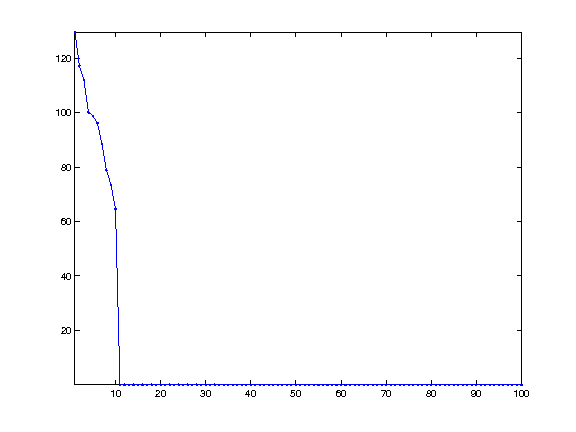## Matrix Completion¶

We consider here a simple measurement operator $\Phi : \RR^{n \times n} \rightarrow \RR^P$ that retains only a sub-set of the entries of the matix. $$\Phi x = ( x_i )_{i \in I}$$ where $\abs{I}=P$ is the set of extracted indexes.

One can of course consider other linear measurement operators.

Number $P$ of measurements.

In :
P = round( n*log(n)*r*1 );


We use here a set of random sampling locations.

In :
I = randperm(n*n); I = I(1:P); I = I(:);


In :
Phi  = @(x)x(I);
PhiS= @(y)reshape( accumarray(I, y, [n*n 1], @sum), [n n]);


Measurement $y=\Phi x_0$.

In :
y = Phi(x0);


The low-rank matrix completion corresponds to the following non-convex minimization. $$x^{\star} \in \uargmin{\Phi x = y} \text{rank}(x).$$

## Noiseless Completion using Douglas Rachford¶

To obtain fast algorithm, it is possible to convexify the objective function and use the nuclear norm $\norm{x}_{\star}$ $$x^{\star} \in \umin{\Phi x = y} \norm{x}_{\star} = \sum_i s_i(x)$$ This is a convex problem, that can be solved efficiently, as we show next.

It is shown in

The Power of Convex Relaxation: Near-Optimal Matrix Completion E. J. Candes and T. Tao, IEEE Trans. Inform. Theory, 56(5), 2053-2080, 2009.

that if the columns of $U(x_0)$ and $V(x_0)$ have a small enough $\ell^\infty$ norm, and if $P \geq C r n \log(n)$ for some absolute constant $C$ then $x^\star=x_0$.

This minimization can be written as $$\umin{ x } F(x) + G(x) \qwhereq \choice{ F(x) = i_{\Cc}(x), \\ G(x) = \norm{x}_{\star}. }$$ where $\Cc = \enscond{x}{\Phi x =y}$.

One can solve this problem using the Douglas-Rachford iterations $$\tilde x_{k+1} = \pa{1-\frac{\mu}{2}} \tilde x_k + \frac{\mu}{2} \text{rPox}_{\gamma G}( \text{rProx}_{\gamma F}(\tilde x_k) ) \qandq x_{k+1} = \text{Prox}_{\gamma F}(\tilde x_{k+1},)$$

We have use the following definition for the proximal and reversed-proximal mappings: $$\text{rProx}_{\gamma F}(x) = 2\text{Prox}_{\gamma F}(x)-x$$ $$\text{Prox}_{\gamma F}(x) = \uargmin{y} \frac{1}{2}\norm{x-y}^2 + \ga F(y).$$

One can show that for any value of $\gamma>0$, any $0 < \mu < 2$, and any $\tilde x_0$, $x_k \rightarrow x^\star$ which is a solution of the minimization of $F+G$.

$$\text{Prox}_{\gamma F}(x) = \uargmin{y} \frac{1}{2}\norm{x-y}^2 + \ga F(y).$$

The proximal operator of $F$ is the orthogonal projection on $\Cc$. It is computed as $$\text{Prox}_{\ga F}(x) = x + \Phi^*(y-\Phi x).$$

In :
ProxF = @(x,gamma)x + PhiS(y-Phi(x));


The proximal operator of $G$ is the soft thresholding of the singular values $$\text{Prox}_{\ga F}(x) = U(x) \rho_\la( S(x) ) V(x)^*$$ where, for $S=\text{diag}(s_i)_i$ $$\rho_\la(S) = \diag\pa{ \max(0,1-\la/\abs{s_i}) s_i }_i.$$

Define $\rho_\la$ as a diagonal operator.

In :
SoftThresh = @(x,gamma)max(0,1-gamma./max(abs(x),1e-10)).*x;


Display it in 1-D.

In :
t = linspace(-10,10,1000);
h = plot(t, SoftThresh(t,3)); axis tight; axis equal;
set(h, 'LineWidth', 2);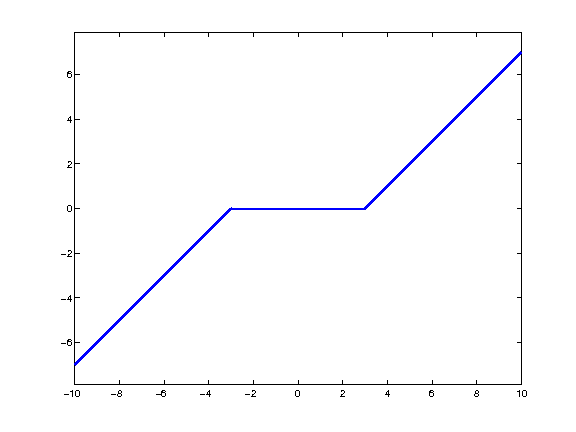Define the proximal mapping $\text{Prox}_{\ga F}$.

In :
prod = @(a,b,c)a*b*c;
SoftThreshDiag = @(a,b,c,gamma)a*diag(SoftThresh(diag(b),gamma))*c';
ProxG = @(x,gamma)apply_multiple_ouput(@(a,b,c)SoftThreshDiag(a,b,c,gamma), @svd, x);


Compute the reversed prox operators.

In :
rProxF = @(x,gamma)2*ProxF(x,gamma)-x;
rProxG = @(x,gamma)2*ProxG(x,gamma)-x;


Value for the $0 < \mu < 2$ and $\gamma>0$ parameters. You can use other values, this might speed up the convergence.

In :
mu = 1;
gamma = 1;


Exercise 1

Implement the Douglas-Rachford iterative algorithm. Keep track of the evolution of the nuclear norm $G(x_k)$.

In :
exo1()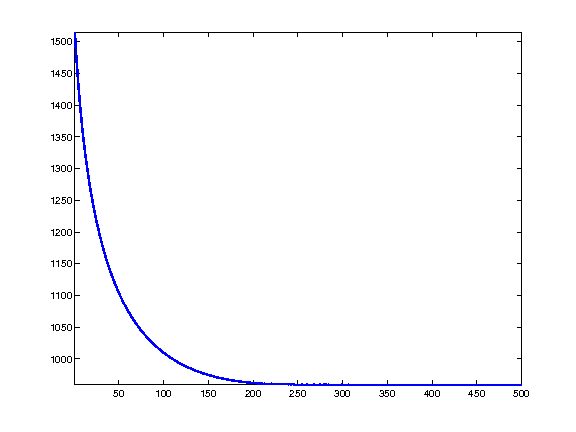In :
%% Insert your code here.


In this case, the matrix is recovered exactly, $A^\star=A_0$.

In :
disp(['|A-A_0|/|A_0| = ' num2str(norm(x-x0)/norm(x), 2)]);

|A-A_0|/|A_0| = 2e-07


Exercise 2

Compute, for several value of rank $r$, an empirical estimate of the ratio of rank-$r$ random matrice than are exactly recovered using nuclear norm minimization.

In :
exo2()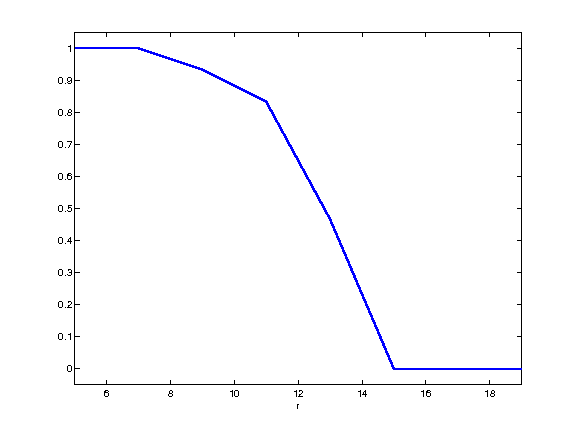In :
%% Insert your code here.


## Noisy Completion using Forward-Backward¶

In the case where $x_0$ does not have low rank but a fast decreasing set of singular values $(s_i(x_0))_i$, and if one has noisy observations $y = \Phi x_0 + w$, where $w \in \RR^P$ is some noise perturbation, then it makes sense to consider a Lagrangian minimization $$\umin{x \in \RR^{n \times n}} \frac{1}{2}\norm{y-\Phi x}^2 + \la \norm{x}_{\star}$$ where $\la>0$ controls the sparsity of the singular values of the solution.

Construct a matrix with decaying singular values.

In :
alpha = 1;
[U,R] = qr(randn(n));
[V,R] = qr(randn(n));
S = (1:n).^(-alpha);
x0 = U*diag(S)*V';


Display the spectrum.

In :
clf;
h = plot(S); axis tight;
set(h, 'LineWidth', 2);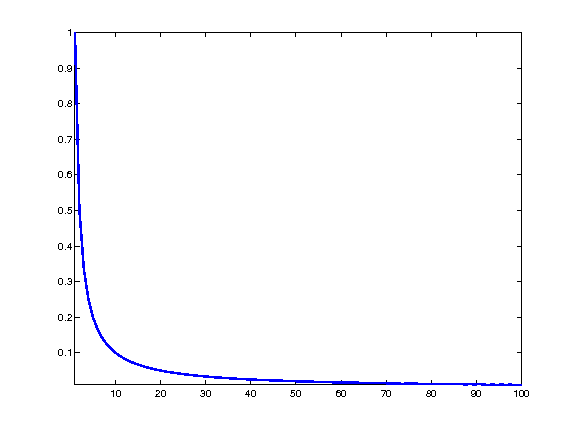Number of measurements.

In :
P = n*n/4;


Measurement operator.

In :
I = randperm(n*n); I = I(1:P); I = I(:);
Phi  = @(x)x(I);
PhiS= @(y)reshape( accumarray(I, y, [n*n 1], @sum), [n n]);


Noise level.

In :
sigma = std(x0(:))/5;


Measurements $y=\Phi x_0 + w$ where $w \in \RR^P$ is a Gaussian white noise.

In :
y = Phi(x0)+sigma*randn(P,1);


It is possible to find a minimizer of the Lagrangian minimization problem using the forward-backward method: $$x_{k+1} = \text{Prox}_{\ga \lambda G}\pa{ x_k - \ga\Phi^*(\Phi x_k - y) }.$$ where $\ga < 2/\norm{\Phi^* \Phi} = 2.$

Value for $\lambda$.

In :
lambda = .01;


Exercise 3

Implement the forward-backward method, monitor the decay of the enrgy minimized by the algorithm.

In :
exo3()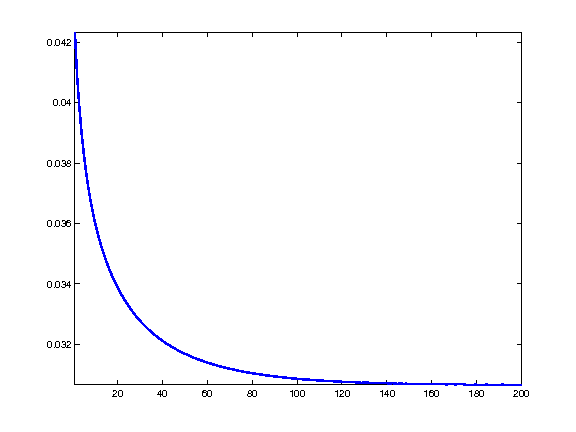In :
%% Insert your code here.


Exercise 4

Plot the error $\norm{x^\star-x_0}/\norm{x_0}$ as a function of the mutiplier $\lambda$.

In :
exo4()

In :
%% Insert your code here.